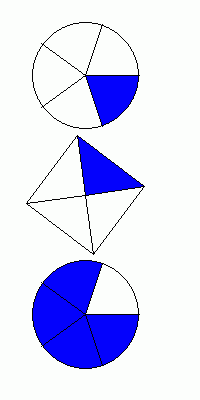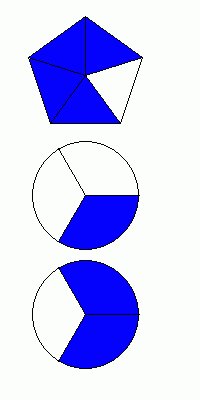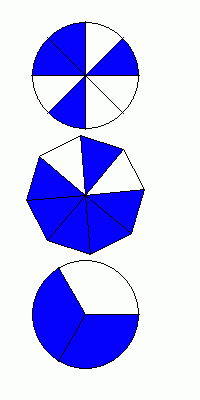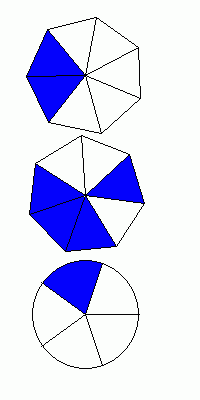# Math Practice Topic: Probability 2

 Description: This topic covers difficult probability problems and teaches you how to compute the odds of an outcome. This is an optional topic. You may need pencil and paper. Adaptive Learning Progression: Numbers get bigger. Start using MathScore for free

## Sample Levels (out of 7)

Find the probability that if you randomly select one piece from each of the three shapes, you will get 3 highlighted pieces and the odds of getting at least one unhighlighted piece.

1.Probability: Odds:

2.Probability: Odds:

Find the probability that if you randomly select one piece from each of the three shapes, you will get 3 highlighted pieces and the odds of getting at least one unhighlighted piece.

1.Probability: Odds:

2.Probability: Odds: